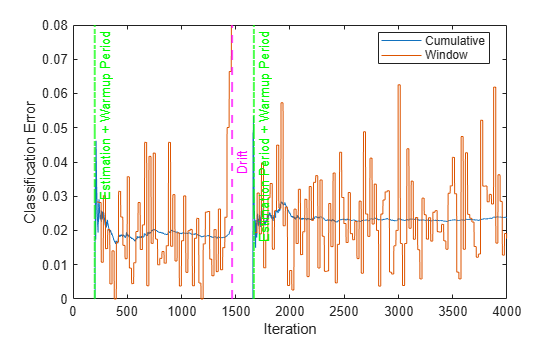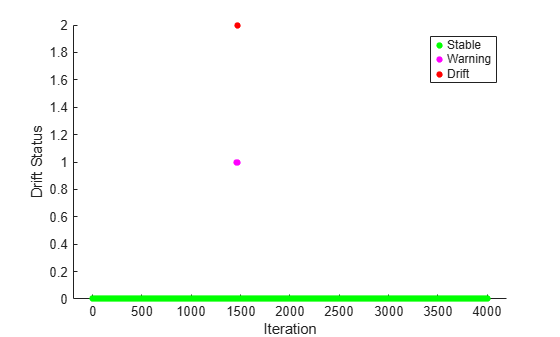# updateMetrics

Update performance metrics in incremental drift-aware learning model given new data

Since R2022b

## Syntax

``Mdl = updateMetrics(Mdl,X,Y)``
``Mdl = updateMetrics(Mdl,X,Y,Name=Value)``

## Description

example

````Mdl = updateMetrics(Mdl,X,Y)` returns an incremental drift-aware learning model `Mdl`, which is the input incremental drift-aware learning model `Mdl` modified to contain the model performance metrics on the incoming predictor and response data, `X` and `Y` respectively.When the input model is warm (`Mdl.IsWarm` is `true`), `updateMetrics` overwrites previously computed metrics, stored in the `Metrics` property, with the new values. Otherwise, `updateMetrics` stores `NaN` values in `Metrics` instead.The input and output models have the same data type.```
````Mdl = updateMetrics(Mdl,X,Y,Name=Value)` uses additional options specified by one or more name-value arguments. For example, you can specify that the columns of the predictor data matrix correspond to observations, and set observation weights.```

## Examples

collapse all

Create the random concept data using the `HelperSineGenerator` and concept drift generator `HelperConceptDriftGenerator`.

```concept1 = HelperSineGenerator("ClassificationFunction",1,"IrrelevantFeatures",true,"TableOutput",false); concept2 = HelperSineGenerator("ClassificationFunction",3,"IrrelevantFeatures",true,"TableOutput",false); driftGenerator = HelperConceptDriftGenerator(concept1,concept2,15000,1000);```

When `ClassificationFunction` is 1, `HelperSineGenerator` labels all points that satisfy x1 < sin(x2) as 1, otherwise the function labels them as 0. When `ClassificationFunction` is 3, this is reversed. That is, `HelperSineGenerator` labels all points that satisfy x1 >= sin(x2) as 1, otherwise the function labels them as 0.

`HelperConceptDriftGenerator` establishes the concept drift. The object uses a sigmoid function `1./(1+exp(-4*(numobservations-position)./width))` to decide the probability of choosing the first stream when generating data . In this case, the position argument is 15000 and the width argument is 1000. As the number of observations exceeds the position value minus half of the width, the probability of sampling from the first stream when generating data decreases. The sigmoid function allows a smooth transition from one stream to the other. Larger width values indicate a larger transition period where both streams are approximately equally likely to be selected.

Initiate an incremental drift-aware model as follows:

1. Create an incremental Naive Bayes classification model for binary classification.

2. Initiate an incremental concept drift detector that uses the Hoeffding's Bounds Drift Detection Method with moving average (HDDMA).

3. Using the incremental linear model and the concept drift detector, instantiate an incremental drift-aware model. Specify the training period as 5000 observations.

```BaseLearner = incrementalClassificationLinear(Solver="sgd"); dd = incrementalConceptDriftDetector("hddma"); idaMdl = incrementalDriftAwareLearner(BaseLearner,DriftDetector=dd,TrainingPeriod=5000);```

Preallocate the number of variables in each chunk and number of iterations for creating a stream of data.

```numObsPerChunk = 10; numIterations = 4000;```

Preallocate the variables for tracking the drift status and drift time, and storing the classification error.

```dstatus = zeros(numIterations,1); statusname = strings(numIterations,1); ce = array2table(zeros(numIterations,2),VariableNames=["Cumulative" "Window"]); driftTimes = [];```

Simulate a data stream with incoming chunks of 10 observations each and perform incremental drift-aware learning. At each iteration:

1. Simulate predictor data and labels, and update the drift generator using the helper function `hgenerate`.

2. Call `updateMetrics` to measure the cumulative performance and the performance within a window of observations. Overwrite the previous incremental model with a new one to track performance metrics.

3. Call `fit` to fit the model to the incoming chunk. Overwrite the previous incremental model with a new one fitted to the incoming observations.

4. Track and record the drift status and the classification error for visualization purposes.

```rng(12); % For reproducibility for j = 1:numIterations % Generate data [driftGenerator,X,Y] = hgenerate(driftGenerator,numObsPerChunk); % Update performance metrics and fit the model idaMdl = updateMetrics(idaMdl,X,Y); idaMdl = fit(idaMdl,X,Y); % Record drift status and classification error statusname(j) = string(idaMdl.DriftStatus); ce{j,:} = idaMdl.Metrics{"ClassificationError",:}; if idaMdl.DriftDetected dstatus(j) = 2; driftTimes(end+1) = j; elseif idaMdl.WarningDetected dstatus(j) = 1; else dstatus(j) = 0; end end```

Plot the cumulative and per window classification error. Mark the warmup and training periods, and where the drift was introduced.

```h = plot(ce.Variables); xlim([0 numIterations]) ylim([0 0.08]) ylabel("Classification Error") xlabel("Iteration") xline((idaMdl.BaseLearner.EstimationPeriod+idaMdl.MetricsWarmupPeriod)/numObsPerChunk,"g-.","Estimation + Warmup Period",LineWidth=1.5) xline((idaMdl.MetricsWarmupPeriod+idaMdl.BaseLearner.EstimationPeriod)/numObsPerChunk+driftTimes,"g-.","Estimation Period + Warmup Period",LineWidth=1.5) xline(driftTimes,"m--","Drift",LabelVerticalAlignment="middle",LineWidth=1.5) legend(h,ce.Properties.VariableNames) legend(h,Location="best")```The plot suggests the following:

• `updateMetrics` computes the performance metrics after the estimation and metrics warm-up period only.

• `updateMetrics` computes the cumulative metrics during each iteration.

• `updateMetrics` computes the window metrics after processing the default metrics window size (200) observations.

• After drift detection `updateMetrics` function waits for `idaMdl.BaseLearner.EstimationPeriod+idaMdl.MetricsWarmupPeriod` observations to start updating model performance metrics again.

Plot the drift status versus the iteration number.

`gscatter(1:numIterations,dstatus,statusname,'gmr','o',4,'on',"Iteration","Drift Status","Filled")`## Input Arguments

collapse all

Incremental drift-aware learning model fit to streaming data, specified as an `incrementalDriftAwareLearner` model object. You can create `Mdl` using the `incrementalDriftAwareLearner` function. For more details, see the object reference page.

Chunk of predictor data to which the model is fit, specified as a floating-point matrix of n observations and `Mdl.BaseLearner.NumPredictors` predictor variables.

When `Mdl.BaseLearner` accepts the `ObservationsIn` name-value argument, the value of `ObservationsIn` determines the orientation of the variables and observations. The default `ObservationsIn` value is `"rows"`, which indicates that observations in the predictor data are oriented along the rows of `X`.

The length of the observation responses (or labels) `Y` and the number of observations in `X` must be equal; `Y(j)` is the response (or label) of observation j (row or column) in `X`.

Note

• If `Mdl.BaseLearner.NumPredictors` = 0, `updateMetrics` infers the number of predictors from `X`, and sets the corresponding property of the output model. Otherwise, if the number of predictor variables in the streaming data changes from `Mdl.BaseLearner.NumPredictors`, `updateMetrics` issues an error.

• `updateMetrics` supports only floating-point input predictor data. If your input data includes categorical data, you must prepare an encoded version of the categorical data. Use `dummyvar` to convert each categorical variable to a numeric matrix of dummy variables. Then, concatenate all dummy variable matrices and any other numeric predictors. For more details, see Dummy Variables.

Data Types: `single` | `double`

Chunk of responses (or labels) to which the model is fit, specified as one of the following:

• Floating-point vector of n elements for regression models, where n is the number of rows in `X`.

• Categorical, character, or string array, logical vector, or cell array of character vectors for classification models. If `Y` is a character array, it must have one class label per row. Otherwise, `Y` must be a vector with n elements.

The length of `Y` and the number of observations in `X` must be equal; `Y(j)` is the response (or label) of observation j (row or column) in `X`.

For classification problems:

• When `Mdl.BaseLearner.ClassNames` is nonempty, the following conditions apply:

• If `Y` contains a label that is not a member of `Mdl.BaseLearner.ClassNames`, `updateMetrics` issues an error.

• The data type of `Y` and `Mdl.BaseLearner.ClassNames` must be the same.

• When `Mdl.BaseLearner.ClassNames` is empty, `updateMetrics` infers `Mdl.BaseLearner.ClassNames` from data.

Data Types: `single` | `double` | `categorical` | `char` | `string` | `logical` | `cell`

### Name-Value Arguments

Specify optional pairs of arguments as `Name1=Value1,...,NameN=ValueN`, where `Name` is the argument name and `Value` is the corresponding value. Name-value arguments must appear after other arguments, but the order of the pairs does not matter.

Example: `ObservationsIn="columns",Weights=W` specifies that the columns of the predictor matrix correspond to observations, and the vector `W` contains observation weights to apply during incremental learning.

Predictor data observation dimension, specified as the comma-separated pair consisting of `'ObservationsIn'` and `'columns'` or `'rows'`.

`updateMetrics` supports `ObservationsIn` only if `Mdl.BaseLearner` supports `ObservationsIn` name-value argument.

Data Types: `char` | `string`

Chunk of observation weights, specified as a floating-point vector of positive values. `updateMetrics` weighs the observations in `X` with the corresponding values in `Weights`. The size of `Weights` must equal n, which is the number of observations in `X`.

By default, `Weights` is `ones(n,1)`.

Example: `Weights=w`

Data Types: `double` | `single`

## Output Arguments

collapse all

Updated incremental drift-aware learning model, returned as an incremental learning model object of the same data type as the input model `Mdl`, `incrementalDriftAwareLearner`.

If the model is not warm, `updateMetrics` does not compute performance metrics. As a result, the `Metrics` property of `Mdl` remains completely composed of `NaN` values. If the model is warm, `updateMetrics` computes the cumulative and window performance metrics on the new data `X` and `Y`, and overwrites the corresponding elements of `Mdl.Metrics`. All other properties of the input model `Mdl` carry over to the output model `Mdl`. For more details, see Performance Metrics.

## Algorithms

collapse all

### Performance Metrics

• The `updateMetrics` and `updateMetricsAndFit` functions track model performance metrics (`Metrics`) from new data when the incremental model is warm (`Mdl.BaseLearner.IsWarm` property). An incremental model becomes warm after `fit` or `updateMetricsAndFit` fits the incremental model to `MetricsWarmupPeriod` observations, which is the metrics warm-up period.

If `Mdl.BaseLearner.EstimationPeriod` > 0, the functions estimate hyperparameters before fitting the model to data. Therefore, the functions must process an additional `EstimationPeriod` observations before the model starts the metrics warm-up period.

• The `Metrics` property of the incremental model stores two forms of each performance metric as variables (columns) of a table, `Cumulative` and `Window`, with individual metrics in rows. When the incremental model is warm, `updateMetrics` and `updateMetricsAndFit` update the metrics at the following frequencies:

• `Cumulative` — The functions compute cumulative metrics since the start of model performance tracking. The functions update metrics every time you call the functions, and base the calculation on the entire supplied data set until a model reset.

• `Window` — The functions compute metrics based on all observations within a window determined by the `MetricsWindowSize` name-value argument. `MetricsWindowSize` also determines the frequency at which the software updates `Window` metrics. For example, if `MetricsWindowSize` is 20, the functions compute metrics based on the last 20 observations in the supplied data (`X((end – 20 + 1):end,:)` and `Y((end – 20 + 1):end)`).

Incremental functions that track performance metrics within a window use the following process:

1. Store `MetricsWindowSize` amount of values for each specified metric, and store the same amount of observation weights.

2. Populate elements of the metrics values with the model performance based on batches of incoming observations, and store the corresponding observation weights.

3. When the window of observations is filled, overwrite `Mdl.Metrics.Window` with the weighted average performance in the metrics window. If the window is overfilled when the function processes a batch of observations, the latest incoming `MetricsWindowSize` observations are stored, and the earliest observations are removed from the window. For example, suppose `MetricsWindowSize` is 20, there are 10 stored values from a previously processed batch, and 15 values are incoming. To compose the length 20 window, the functions use the measurements from the 15 incoming observations and the latest 5 measurements from the previous batch.

• The software omits an observation with a `NaN` prediction (score for classification and response for regression) when computing the `Cumulative` and `Window` performance metric values.

### Observation Weights

For classification problems, if the prior class probability distribution is known (in other words, the prior distribution is not empirical), `updateMetrics` normalizes observation weights to sum to the prior class probabilities in the respective classes. This action implies that observation weights are the respective prior class probabilities by default.

For regression problems or if the prior class probability distribution is empirical, the software normalizes the specified observation weights to sum to 1 each time you call `updateMetrics`.

## Version History

Introduced in R2022b